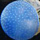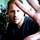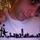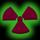## General Question# Do you know of any website that can solve quadratic inequalities?

Asked by Magnus (2871) May 19th, 2008

I have a math-test tomorrow and I need to know the answer of this to understand. What interval of numbers for X can be used for X(squared)-X-4 = or > 0 ? And I’m really sorry if some of the terms are wrong, but I hope you can understand me.

Observing members: 0Composing members: 0Been a while since I’ve done math… I think the result set would be the unbounded interval (-∞, ∞) a.k.a the set of all real numbers.

But a web site to tell you that? Hmmmm…

They’d likely be running something like Maple or Mathematica.

I also would be interested if anyone turns something up.

: you could write your equation as: x^2 – x – 4 >= 0

robmandu (21285)“Great Answer” (0) Flag as…you could easily set it up at sagenb.com if you feel like learning sage. But you’d have to do the programming.

finkelitis (1907)“Great Answer” (0) Flag as…The answer is either <<-, -1.56]u[2,56, ->> or [-1.56]u[2.56, ->> .

Magnus (2871)“Great Answer” (0) Flag as…Solve the quadratic inequality as you would do it if it were equal to 0. The values you get will be potential solutions, but if you evaluate the function at one and it you get something like 2 > 3, then you know that that value isn’t a solution.

For example if you had x^2 + 3 < -1, solve it as you would x^2 + 3 = -1. You would end up with x^2 +4 =0. Solve for x you get (x +2)^2 so your value for x in this case is ±2. Plug the values into the original x^2 + 3 < -1 and you will get (2)^2 +3 < -1 and (-2)^2 + 3 < -1. You will get 7 < -1 for both solutions, and since 7 is not less than -1, then there is no solution for that inequality.

winblowzxp (498)“Great Answer” (0) Flag as…Solve the inequality: f(x) = x^2 – x – 4 > 0. (or = 0)
Solution. Use the quadratic formula to solve f(x) = 0. It gives as 2 real roots: -1.56 and 2.56. Plot these 2 values on the number line. Use the origin as test point. Substitute x = 0 into the inequality. you get -4 > 0. It is not TRUE! Then, the origine is located on the “wrong” segment. The answers should be the 2 rays. The solution set are the two half closed intervals: (-infinity, -1.56] and [2.56 , +infinity). This means the two end points -1. 56 and 2.56 are included in the solution set.
There are many websites that explain how to solve quadratic inequalities. Just search into Google.com and type in “Solving quadratic inequalities”.

1350ng (1)“Great Answer” (0) Flag as…or